Next: WHITTLE'S EXP-LOG METHOD Up: Spectral factorization Previous: ROBINSON'S ENERGY DELAY THEOREM

# THE TOEPLITZ METHOD

The Toeplitz method of spectral factorization is based on special properties of Toeplitz matrices In this chapter we introduce the Toeplitz matrix to perform spectral factorization. In later chapters we will refer back several times to the algebra described here. When one desires to predict a time series, one can do this with a so-called prediction filter. This filter is found as the solution to Toeplitz simultaneous equations. Norman Levinson, in his explanatory appendix of Norbert Wiener's Time Series, first introduced the Toeplitz matrix to engineers; however, it had been widely known and used previously in the field of econometrics. It is only natural that it should appear first in economics because there the data are observed at discrete time points, whereas in engineering the idea of discretized time was rather artificial until the advent of digital computers. The need for prediction in economics is obvious. In seismology, it is not the prediction itself but the error in prediction which is of interest. Reflection seismograms are used in petroleum exploration. Ideally, the situation is like radar where the delay time is in direct proportion to physical distance. This is the case for the so-called primary reflections. A serious practical complication arises in shallow seas where large acoustic waves bounce back and forth between the sea surface and the sea floor. These are called multiple reflections. A mechanism for separation of the primary waves from the multiple reflections is provided by prediction. A multiple reflection is predictable from earlier echoes, but a primary reflection is not predictable from earlier echoes. Thus, the useful information is carried in the part of the seismogram which is not predictable. An oil company computer devoted to interpreting seismic exploration data typically solves about 100,000 sets of Toeplitz simultaneous equations in a day.

Another important application of the algebra associated with Toeplitz matrices is in high-resolution spectral analysis. This is where a power spectrum is to be estimated from a sample of data which is short (in time or space). The conventional statistical and engineering knowledge in this subject is based on assumptions which are frequently inappropriate in geophysics. The situation was fully recognized by John P. Burg who utilized some of the special properties of Toeplitz matrices to develop his maximum-entropy spectral estimation procedure described in a later chapter.

Another place where Toeplitz matrices play a key role is in the mathematical physics which describes layered materials. Geophysicists often model the earth by a stack of plane layers or by concentric spherical shells where each shell or layer is homogeneous. Surprisingly enough, many mathematical physics books do not mention Toeplitz matrices. This is because they are preoccupied with forward problems; that is, they wish to calculate the waves (or potentials) observed in a known configuration of materials. In geophysics, we are interested in both forward problems and in inverse problems where we observe waves on the surface of the earth and we wish to deduce material configurations inside the earth. A later chapter contains a description of how Toeplitz matrices play a central role in such inverse problems.

We start with a time function xt which may or may not be minimum phase. Its spectrum is computed by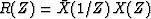.As we saw in the preceding sections, given R(Z) alone there is no way of knowing whether it was computed from a minimum-phase function or a nonminimum-phase function. We may suppose that there exists a minimum phase B(Z) of the given spectrum, that is,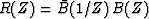.Since B(Z) is by hypothesis minimum phase, it has an inverse A(Z) = 1/B(Z). We can solve for the inverse A(Z) in the following way: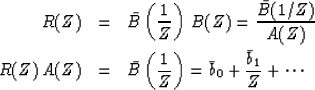(7) (8)
To solve for A(Z), we identify coefficients of powers of Z. For the case where, for example, A(Z) is the quadratic a0 + a1 Z + a2 Z2, the coefficient of Z0 in (8) is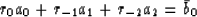(9)
The coefficient of Z1 is

 r1 a0 + r0 a1 + r-1 a2 = 0 (10)

and the coefficient of Z2 is

 r2 a0 + r1 a1 + r0 a2 = 0 (11)

Bring these together we have the simultaneous equations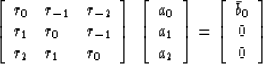(12)
It should be clear how to generalize this to a set of simultaneous equations of arbitrary size. The main diagonal of the matrix contains r0 in every position. The diagonal just below the main one contains r1 everywhere. Likewise, the whole matrix is filled. Such a matrix is called a Toeplitz matrix. Let us define a'k = ak/a0. Recall by the polynomial division algorithm that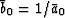.Define a positive number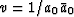.Now, dividing the vector on each side of (12) by a0, we get the most popular form of the equations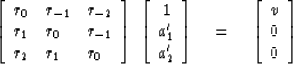(13)
This gives three equations for the three unknowns a'1, a'2, and v. To put (13) in a form where standard simultaneous equations programs could be used one would divide the vectors on both sides by v. After solving the equations, we get a0 by noting that it has magnitude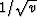and its phase is arbitrary, as with the root method of spectral factorization.

At this point, a pessimist might interject that the polynomial A(Z) = a0 + a1 Z + a2 Z2 determined from solving the set of simultaneous equations might not turn out to be minimum phase, so that we could not necessarily compute B(Z) by B(Z) = 1/A(Z). The pessimist might argue that the difficulty would be especially likely to occur if the size of the set (13) was not taken to be large enough. Actually experimentalists have known for a long time that the pessimists were wrong. A proof can now be performed rather easily, along with a description of a computer algorithm which may be used to solve (13).

The standard computer algorithms for solving simultaneous equations require time proportional to n3 and computer memory proportional to n2. The Levinson computer algorithm for Toeplitz matrices requires time proportional to n2 and memory proportional to n. First notice that the Toeplitz matrix contains many identical elements. Levinson utilized this special Toeplitz symmetry to develop his fast method.

The method proceeds by the approach called recursion. That is, given the solution to the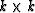set of equations, we show how to calculate the solution to the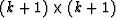set. One must first get the solution for k = 1; then one repeatedly (recursively) applies a set of formulas increasing k by one at each stage. We will show how the recursion works for real-time functions (rk = r-k) going from the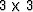set of equations to the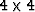set, and leave it to the reader to work out the general case.

Given thesimultaneous equations and their solution a1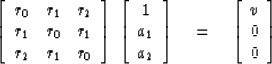(14)
then the following construction defines a quantity e given r3 (or r3 given e)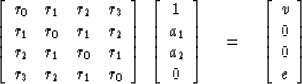(15)
The first three rows in (15) are the same as (14); the last row is the new definition of e. The Levinson recursion shows how to calculate the solution a' to thsimultaneous equations which is like (14) but larger in size.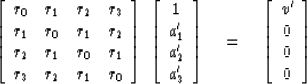(16)

The important trick is that from (15) one can write a reversed'' system of equations. (If you have trouble with the matrix manipulation, merely write out (16) as simultaneous equations, then reverse the order of the unknowns, and then reverse the order of the equations.)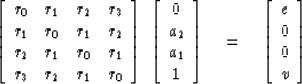(17)
The Levinson recursion consists of subtracting a yet unknown portion c3 of (17) from (15) so as to get the result (16). That is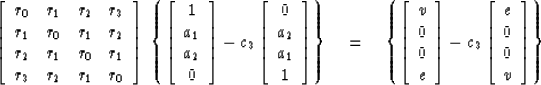(18)
To make the right-hand side of (18) look like the right-hand side of (3-3-8), we have to get the bottom element to vanish, so we must choose c3 = e/v. This implies that v' = v - c3 e = v - e2/v = v[1 - (e/v)2]. Thus, the solution to thesystem is derived from theby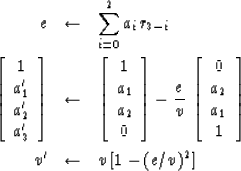(19) (20) (21)

We have shown how to calculate the solution of theToeplitz equations from the solution of theToeplitz equations. The Levinson recursion consists of doing this type of step, starting from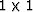and working up to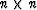.

       COMPLEX R,A,C,E,BOT,CONJG
C(1)=-1.; R(1)=1.; A(1)=1.; V(1)=1.
200  DO 220 J=2,N
A(J)=0.
E=0.
DO 210 I=2,J
210  E=E+R(I)*A(J-I+1)
C(J)=E/V(J-1)
V(J)=V(J-1)-E*CONJG(C(J))
JH=(J+1)/2
DO 220 I=1,JH
BOT=A(J-I+1)-C(J)*CONJG(A(I))
A(I)=A(I)-C(J)*CONJG(A(J-I+1))
220  A(J-I+1)=BOT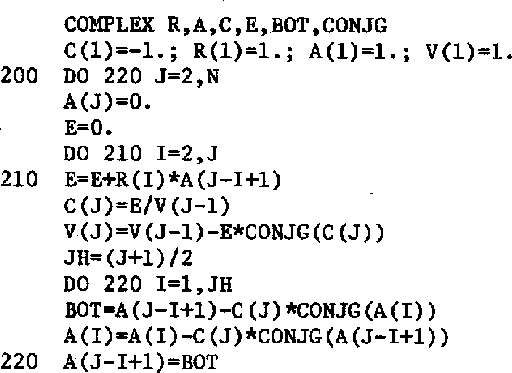3-3
Figure 3
A computer program to do the Levinson recursion. It is assumed that the input rk have been normalized by division by r0. The complex arithmetic is optional.Let us reexamine the calculation to see why A(Z) turns out to be minimum phase. First, we notice that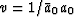andare always positive. Then from (21) we see that -1 < e/v < +1. (The fact that c = e/v is bounded by unity will later be shown to correspond to the fact that reflection coefficients for waves are so bounded.) Next, (20) may be written in polynomial form as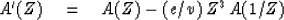(22)
We know that Z3 has unit magnitude on the unit circle. Likewise (for real time series), the spectrum of A(Z) equals that of A(1/Z). Thus (by the theorem of adding garbage to a minimum-phase wavelet) if A(Z) is minimum phase, the A'(Z) will also be minimum phase. In summary, the following three statements are equivalent:

1. R(Z) is of the form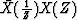.

2. |ck| < 1.

3. A(Z) is minimum phase.

If any one of the above three is false, then they are all false. A program for the calculation of ak and ck from rk is given in Figure 3. In Chapter 8, on wave propagation in layers, programs are given to compute rk from ak or ck.

## EXERCISES:

1. The top row of aToeplitz set of simultaneous equations like (16) is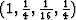.What is the solution ak?
2. How must the Levinson recursion be altered if time functions are complex? Specifically, where do complex conjugates occur in (19), (8), and (21)?
3. Let Am(Z) denote a polynomial whose coefficients are the solution to an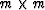set of Toeplitz equations. Show that if Bk(Z) = Zk Ak(Z-1) then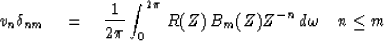which means that the polynomial Bm(Z) is orthogonal to polynomial Zn over the unit circle under the positive weighting function R. Utilizing this result, state why Bm is orthogonal to Bn, that is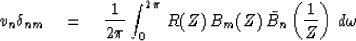(HINT:  First consider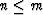, then all n.)

Toeplitz matrices are found in the mathematical literature under the topic of polynomials orthogonal on the unit circle. The author especially recommends Atkinson's book.Next: WHITTLE'S EXP-LOG METHOD Up: Spectral factorization Previous: ROBINSON'S ENERGY DELAY THEOREM
Stanford Exploration Project
10/30/1997